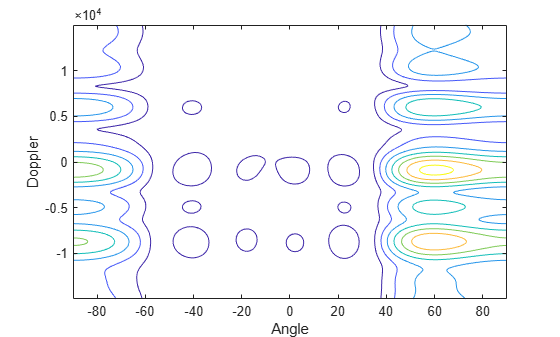Documentation

# phased.AngleDopplerResponse

Angle-Doppler response

## Description

The `AngleDopplerResponse` object calculates the angle-Doppler response of input data.

To compute the angle-Doppler response:

1. Define and set up your angle-Doppler response calculator. See Construction.

2. Call `step` to compute the angle-Doppler response of the input signal according to the properties of `phased.AngleDopplerResponse`. The behavior of `step` is specific to each object in the toolbox.

### Note

Starting in R2016b, instead of using the `step` method to perform the operation defined by the System object™, you can call the object with arguments, as if it were a function. For example, ```y = step(obj,x)``` and `y = obj(x)` perform equivalent operations.

## Construction

`H = phased.AngleDopplerResponse` creates an angle-Doppler response System object, `H`. This object calculates the angle-Doppler response of the input data.

`H = phased.AngleDopplerResponse(Name,Value)` creates angle-Doppler object, `H`, with each specified property Name set to the specified Value. You can specify additional name-value pair arguments in any order as (`Name1`,`Value1`,...,`NameN`,`ValueN`).

## Properties

`SensorArray`

Sensor array

Sensor array specified as an array System object belonging to the `phased` package. A sensor array can contain subarrays.

Default: `phased.ULA` with default property values

`PropagationSpeed`

Signal propagation speed

Specify the propagation speed of the signal, in meters per second, as a positive scalar. You can specify this property as single or double precision.

Default: Speed of light

`OperatingFrequency`

System operating frequency

Specify the operating frequency of the system in hertz as a positive scalar. The default value corresponds to 300 MHz. You can specify this property as single or double precision.

Default: `3e8`

`PRFSource`

Source of PRF values

Source of the PRF values for the STAP processor, specified as `'Property'` or `'Input port'`. When you set this property to `'Property'`, the PRF is determined by the value of the `PRF` property. When you set this property to ```'Input port'```, the PRF is determined by an input argument to the `step` method at execution time.

Default: `'Property'`

`PRF`

Pulse repetition frequency

Specify the pulse repetition frequency (PRF) in hertz of the input signal as a positive scalar. This property applies when you set the `PRFSource` property to `'Property'`. You can specify this property as single or double precision.

Default: `1`

`ElevationAngleSource`

Source of elevation angle

Specify whether the elevation angle comes from the `ElevationAngle` property of this object or from an input argument in `step`. Values of this property are:

 `'Property'` The `ElevationAngle` property of this object specifies the elevation angle. `'Input port'` An input argument in each invocation of `step` specifies the elevation angle.

Default: `'Property'`

`ElevationAngle`

Elevation angle

Specify the elevation angle in degrees used to calculate the angle-Doppler response as a scalar. The angle must be between –90 and 90. This property applies when you set the `ElevationAngleSource` property to `'Property'`. You can specify this property as single or double precision.

Default: `0`

`NumAngleSamples`

Number of samples in angular domain

Specify the number of samples in the angular domain used to calculate the angle-Doppler response as a positive integer. This value must be greater than 2. You can specify this property as single or double precision.

Default: `256`

`NumDopplerSamples`

Number of samples in Doppler domain

Specify the number of samples in the Doppler domain used to calculate the angle-Doppler response as a positive integer. This value must be greater than 2. You can specify this property as single or double precision.

Default: `256`

## Methods

 plotResponse Plot angle-Doppler response step Calculate angle-Doppler response
Common to All System Objects
`release`

Allow System object property value changes

## Examples

expand all

Calculate the angle-Doppler response of the 190th cell of a collected data cube.

Load data cube and construct a `phased.AngleDopplerResponse` System object™.

```load STAPExampleData; x = shiftdim(STAPEx_ReceivePulse(190,:,:)); response = phased.AngleDopplerResponse(... 'SensorArray',STAPEx_HArray,... 'OperatingFrequency',STAPEx_OperatingFrequency,... 'PropagationSpeed',STAPEx_PropagationSpeed,... 'PRF',STAPEx_PRF);```

Plot angle-Doppler response.

```[resp,ang_grid,dop_grid] = response(x); contour(ang_grid,dop_grid,abs(resp)) xlabel('Angle') ylabel('Doppler')```expand all

## References

 Guerci, J. R. Space-Time Adaptive Processing for Radar. Boston: Artech House, 2003.